Loading World Series Futures...DraftKings World Series Odds
+350
3.5 to 1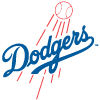Dodgers
17.9% implied probability

+650
6.5 to 1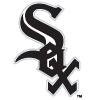White Sox
10.7% implied probability

+700
7 to 1Padres
10.1% implied probability

+950
9.5 to 1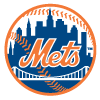Mets
7.7% implied probability

+1200
12 to 1Yankees
6.2% implied probability

+1600
16 to 1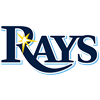Rays
4.7% implied probability

+1600
16 to 1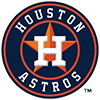Astros
4.7% implied probability

+2000
20 to 1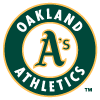Athletics
3.8% implied probability

+2100
21 to 1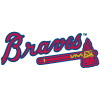Braves
3.7% implied probability

+2200
22 to 1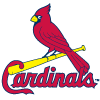Cardinals
3.5% implied probability

+2200
22 to 1Red Sox
3.5% implied probability

+2500
25 to 1Blue Jays
3.1% implied probability

+2500
25 to 1Brewers
3.1% implied probability

+3300
33 to 1Giants
2.4% implied probability

+4000
40 to 1Indians
2.0% implied probability

+4000
40 to 1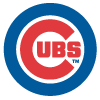Cubs
2.0% implied probability

+5000
50 to 1Phillies
1.6% implied probability

+6000
60 to 1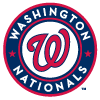Nationals
1.3% implied probability

+7000
70 to 1Marlins
1.1% implied probability

+7000
70 to 1Angels
1.1% implied probability

+7000
70 to 1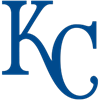Royals
1.1% implied probability

+7000
70 to 1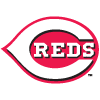Reds
1.1% implied probability

+8000
80 to 1Twins
1.0% implied probability

+10000
100 to 1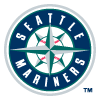Mariners
0.8% implied probability

+25000
250 to 1Tigers
0.3% implied probability

+25000
250 to 1Rockies
0.3% implied probability

+25000
250 to 1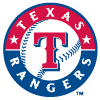Rangers
0.3% implied probability

+25000
250 to 1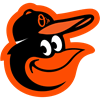Orioles
0.3% implied probability

+25000
250 to 1Diamondbacks
0.3% implied probability

+30000
300 to 1Pirates
0.3% implied probability